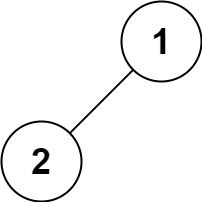## 144. Binary Tree Preorder Traversal

Given the `root` of a binary tree, return the preorder traversal of its nodes' values.

Example 1:```Input: root = [1,null,2,3]
Output: [1,2,3]
```

Example 2:

```Input: root = []
Output: []
```

Example 3:

```Input: root = 
Output: 
```

Example 4:```Input: root = [1,2]
Output: [1,2]
```

Example 5:```Input: root = [1,null,2]
Output: [1,2]
```

Constraints:

• The number of nodes in the tree is in the range `[0, 100]`.
• `-100 <= Node.val <= 100`

Follow up: Recursive solution is trivial, could you do it iteratively?

## Rust Solution

``````struct Solution;

trait Preorder {
fn preorder(&self, all: &mut Vec<i32>);
}

fn preorder(&self, all: &mut Vec<i32>) {
if let Some(node) = self {
let node = node.borrow();
all.push(node.val);
node.left.preorder(all);
node.right.preorder(all);
}
}
}

impl Solution {
fn preorder_traversal(root: TreeLink) -> Vec<i32> {
let mut res = vec![];
root.preorder(&mut res);
res
}
}

#[test]
fn test() {
let root = tree!(1, None, tree!(2, tree!(3), None));
let res = vec![1, 2, 3];
assert_eq!(Solution::preorder_traversal(root), res);
}
``````

Having problems with this solution? Click here to submit an issue on github.